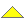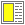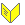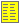Test of Object-oriented Programs - slide 16 : 35

Example of Equivalence Partitioning (1)
```  // Exchange element i and j in table
public void SwapElements<T>(T[] table, int i, int j){
...
}   ```
• Equivalence partitions - all combinations of

• table

• table is empty, table is singular, table with two or more elements

• i and j

• One of i and j are outside the bounds of table

• i inside and j outside, i outside and j inside, both are outside

• Both i and j are inside the bounds of table

• i < j, i > j, i = j

Use of a stronger precondition limits the number of test cases